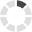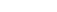### Site InformationLoading... Please wait...

# MATH125 Final Exam (APUS)

\$25.00

## Product Description

MATH125 Final Exam (APUS)

Question 1 of 25

1.0/ 1.0 Points

The length of a garden is double its width. There is a fence around the perimeter that measures 228 ft. What are the length and width of the garden?

A.length = 40 ft, width = 80 ft

B.length = 80 ft, width = 40 ft

C.length = 76 ft, width = 38 ft

D.length = 38 ft, width = 76 ft

Question 2 of 25

1.0/ 1.0 Points

Let U = [5, 10, 15, 20, 25, 30, 35, 40] A = [5, 10, 15, 20] B = [25, 30, 35, 40] C = [10, 20, 30, 40]. Find A U B.

A.A U B = [10, 15]

B.A U B = Ø

C.A U B = [5,10, 15, 20, 25]

D.A U B = [5, 10, 15, 20, 25, 30, 35, 40]

Question 3 of 25

1.0/ 1.0 Points

The table shows the students from Genius High School with the four highest GPAs from 2005 to 2007. Write the region(s) of the Venn diagram that would include Kellyn. (Note set X represents 2005 top-ranked students, set Y represents 2006 top-ranked students, and set Z represents 2007 top-ranked students).

A.Region I

B.Region III

C.Region V

D.Region IV

Question 4 of 25

1.0/ 1.0 Points

A person sold their house for \$150,000 and made a profit of 37 percent. How much did they pay for their home?

A.\$55,500.00

B.\$171,250.96

C.\$135,000.79

D.\$109,489.05

Question 5 of 25

1.0/ 1.0 Points

Gail is trying to stay between 475 calories and 675 calories for lunch. She selects a sandwich for 325 calories and a salad for 107 calories. How many calories can she have for dessert?

A.Between 43 and 275 calories

B.Between 43 and 243 calories

C.Between 100 and 275 calories

D.Between 100 and 243 calories

Question 6 of 25

1.0/ 1.0 Points

Adult tickets for a play cost \$11 and child tickets cost \$5. If there were 30 people at a performance and the theatre collected \$198 from ticket sales, how many children attended the play?

A.22 children

B.21 children

C.23 children

D.8 children

Question 7 of 25

1.0/ 1.0 Points

Graph the linear function: f(x) = -2x + 4

B.

C.

D.

Question 8 of 25

1.0/ 1.0 Points

The total real estate commission for a real estate company was \$60 million in 2008, an increase of \$25 million over the year 2004. What was the percent increase? Round answer to the nearest tenth of a percent.

A.28.6 percent

B.71.4 percent

C.41.7 percent

D.58.3 percent

Question 9 of 25

1.0/ 1.0 Points

Dr. Sand borrowed some money to buy new furniture for her office. She paid \$634.41 simple interest on a 3.5-year loan at 4.2 percent. Find the principal.

A.\$93.26

B.\$4,315.71

C.\$2,220.44

D.\$5,000.00

Question 10 of 25

1.0/ 1.0 Points

In order to help pay for college, the grandparents of a child invest \$2,500 in a bond that pays 8 percent interest compounded quarterly. How much money will there be in 4 years?

A.\$3,542.10

B.\$3,521.72

C.\$3,431.96

D.\$3,401.60

Question 11 of 25

1.0/ 1.0 Points

Katie had an unpaid balance of \$3,155.15 on her credit card statement at the beginning of October. She made a payment of \$215.00 during the month, and made purchases of \$412.01. If the interest rate on Katie's credit card was 6.5 percent per month on the unpaid balance, find her finance charge and the new balance on November 1.

A.Finance charge = \$200.00; new balance = \$3,452.17

B.Finance charge = \$205.08; new balance = \$3,557.25

C.Finance charge = \$215.19; new balance = \$3,467.36

D.Finance charge = \$195.14; new balance = \$3,447.31

Question 12 of 25

1.0/ 1.0 Points

Use an English/Metric conversion for area given in the table to convert the following measurement to the specified measurement. Round to the nearest hundredth, if necessary.

1 in2 ≈ 6.45 cm2

1 ft2 ≈ 0.093 m2

1 yd2 ≈ 0.836 m2

1 mi2 ≈ 2.59 km2

1 acre ≈ 4,047 m2

10,427 in2 = _____ dm2

A.161,409.60 dm2

B.10.42 dm2

C.6,725,400 dm2

D.672.54 dm2

Question 13 of 25

1.0/ 1.0 Points

Convert the following Celsius temperature to an equivalent Fahrenheit temperature. –5 degrees C

A.0 degrees F

B.7 degrees F

C.–1 degrees F

D.23 degrees F

Question 14 of 25

1.0/ 1.0 Points

Find the perimeter.

A.35 inches

B.25 inches

C.48 inches

D.45 inches

Question 15 of 25

1.0/ 1.0 Points

The triangles in the figure below are similar. Use the proportional property of similar triangles to find the measure of x.

A.34.2 km

B.29.2 km

C.21 km

D.19.2 km

Question 16 of 25

1.0/ 1.0 Points

Find the area.

A.900 sq mi

B.240 sq mi

C.150 sq mi

D.450 sq mi

Question 17 of 25

1.0/ 1.0 Points

Suppose the cone below has a radius of 7inches and a height of 10 inches. Find the volume of the cone.

Round to two decimal places.

A.339.29 cu in

B.1,526.81 cu in

C.512.87 cu in

D.226.19 cu in

Question 18 of 25

1.0/ 1.0 Points

In a classroom, the students are 12 boys and 6 girls. If one student is selected at random, find the probability that the student is a girl.

A.2/3

B.1/2

C.2/9

D.1/3

Question 19 of 25

1.0/ 1.0 Points

To enter a contest, a person must select 7 numbers from 49 numbers. In order to win a prize one of the numbers must match. Find the probability of winning if a person buys one ticket. (Note: The numbers can be selected in any order and any one of the seven winning numbers on the ticket is a win.)

A.0.001

B.0.0167

C.0.14

D.0.0007

Question 20 of 25

1.0/ 1.0 Points

A single card is drawn from a deck. Find the probability of selecting a 4 or a club.

A.7/52

B.9/26

C.4/13

D.17/52

Question 21 of 25

1.0/ 1.0 Points

Twenty marbles are used to spell a word—9 blue ones, 5 red ones, 3 yellow ones and 3 green ones. If two marbles are drawn from the set of twenty marbles at random in succession and without replacement, what is the probability (as a reduced fraction) of choosing a marble other than green each time?

A.1/11

B.3/17

C.1/3

D.68/95

Question 22 of 25

1.0/ 1.0 Points

Find the mean, median, mode, and midrange for the data provided. The data shows hours spent at work for a group of men.

A.mean: 65, median: 67.575, mode: 65, midrange: 30.6

B.mean: 65, median: 67.575, mode: 67, midrange: 30.6

C.mean: 67.575, median: 65, mode: no mode, midrange: 67

D.mean: 67.575, median: 67, mode: no mode, midrange: 67

Question 23 of 25

1.0/ 1.0 Points

Assume a data set is normally distributed with mean 121 and standard deviation 15. If the data set contains 650 data values, approximately how many of the data values will fall within the range 91 to 151?

A.574

B.620

C.318

D.424

Question 24 of 25

1.0/ 1.0 Points

The average amount customers at a certain grocery store spend yearly is \$665.98. Assume the variable is normally distributed. If the standard deviation is \$93.27, find the probability that a randomly selected customer spends between \$638.00 and \$829.20.

A.0.330 = 33.0 percent

B.0.701 = 70.1 percent

C.0.578 = 57.8 percent

D.0.342 = 34.2 percent

Question 25 of 25

0.0/ 1.0 Points

In a class of 300 students, John’s rank was 40. Find his percentile rank.

A.26

B.90

C.13

D.87

## Customers also viewed

• \$25.00• \$25.00• \$25.00• \$25.00• \$25.00## Related Products

• CHFD212 Final Exam (APUS) \$25.00• CHFD311 Final Exam (APUS) \$25.00• LSTD207 Final Exam (APUS) \$25.00• LSTD209 Final Exam (APUS) \$25.00• SCIN138 Final Exam (APUS) \$30.00Click the button below to add the MATH125 Final Exam (APUS) to your wish list.